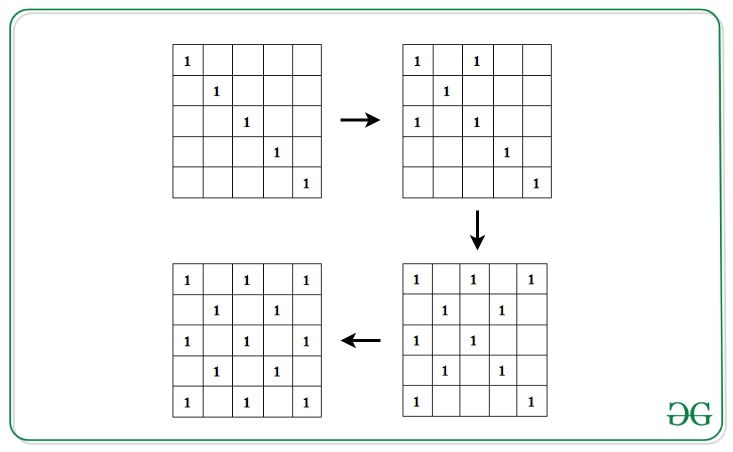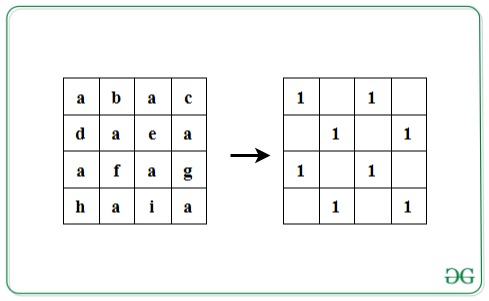# Check if given string satisfies the following conditions

• Last Updated : 02 Dec, 2021

Given a string S of length L, where L is a perfect square, the task is to check if the given string satisfies the following conditions:

• Insert the characters of the string into a square matrix A[][] of dimensions √L x √L in row-wise manner.
• Initialize another matrix M[][] with 0s. Fill the left diagonal with 1. Now, for 1 present in the left diagonal, fill its corresponding right diagonal with 1s.• Now, check if all the indices in matrix M[][] which contains 1, contains the same character in A[][].

If the condition is satisfied, print “Yes”. Otherwise, print “No”.

Examples:

Input: S = ”abacdaeaafaghaia”
Output: Yes
Explanation:Input: S = ”abacdaeabfaghaia”
Output: No

Approach: The idea is to traverse the matrix A[][] where its corresponding character in the matrix M[][] is 1. Follow the steps below to solve the problem:

• Calculate the dimensions of the matrix as N = √L.
• Iterate over the left diagonal by visiting each cell, A[i][i] where 1<= i<= N.
• For every element of the left diagonal at cell A[i][i], initialize variables x and y with i and traverse its corresponding right diagonal by visiting the character S[x*N + y] and S[y*N + x] and decrement x each time by 1 and increment y each time by 1 to move along the next cells in the right diagonals, while x is not smaller than 0 and y is smaller than N.
• If all characters are found to be same in the above step, print “Yes”. Otherwise, print “No” if any mismatch is found.

Below is the implementation of the above approach:

## C++

 `// C++ program for the above approach`   `#include ` `using` `namespace` `std;`   `// Function to check if given string` `// satisfies the given conditions` `void` `isValid(string s)` `{`   `    ``// Dimensions` `    ``int` `n = ``sqrt``(s.length());`   `    ``char` `check = s;`   `    ``// Left diagonal` `    ``for` `(``int` `i = 0; i < n; i++) {` `        ``int` `x = i, y = i;`   `        ``// Right diagonal` `        ``while` `(x >= 0 && y < n) {` `            ``if` `(s[(n * x) + y] != check` `                ``|| s[(n * y) + x] != check) {`   `                ``// Conditions not satisfied` `                ``cout << ``"No"` `<< endl;` `                ``return``;` `            ``}` `            ``x--;` `            ``y++;` `        ``}` `    ``}`   `    ``// Print Yes` `    ``cout << ``"Yes"` `<< endl;` `}`   `// Driver Code` `int` `main()` `{`   `    ``// Given String` `    ``string str = ``"abacdaeaafaghaia"``;`   `    ``// Function call` `    ``isValid(str);`   `    ``return` `0;` `}`

## Java

 `// Java program for the above approach` `import` `java.util.*;` `  `  `class` `GFG{` `  `  `// Function to check if given string` `// satisfies the given conditions` `static` `void` `isValid(String s)` `{` `    `  `    ``// Dimensions` `    ``int` `n = (``int``)Math.sqrt(s.length());` `     `  `    ``char` `check = s.charAt(``0``);` `     `  `    ``// Left diagonal` `    ``for``(``int` `i = ``0``; i < n; i++)` `    ``{` `        ``int` `x = i, y = i;` `        `  `        ``// Right diagonal` `        ``while` `(x >= ``0` `&& y < n)` `        ``{` `            ``if` `(s.charAt((n * x) + y) != check ||` `                ``s.charAt((n * y) + x) != check) ` `            ``{` `                `  `                ``// Conditions not satisfied` `                ``System.out.print(``"No"``);` `                ``return``;` `            ``}` `            ``x--;` `            ``y++;` `        ``}` `    ``}` `    `  `    ``// Print Yes` `    ``System.out.print(``"Yes"``);` `} ` `  `  `// Driver Code` `public` `static` `void` `main(String[] args)` `{` `    `  `    ``// Given String` `    ``String str = ``"abacdaeaafaghaia"``;` `    `  `    ``// Function call` `    ``isValid(str);` `}` `}`   `// This code is contributed by code_hunt`

## Python3

 `# Python3 program for the above approach` `import` `math`   `# Function to check if given string` `# satisfies the given conditions` `def` `isValid(s):` `    `  `    ``# Dimensions` `    ``n ``=` `int``(math.sqrt(``len``(s)))` `    ``check ``=` `s[``0``]`   `    ``# Left diagonal` `    ``for` `i ``in` `range``(n):` `        ``x ``=` `i` `        ``y ``=` `i` `        `  `        ``# Right diagonal` `        ``while` `(x >``=` `0` `and` `y < n):` `            ``if` `(s[n ``*` `x ``+` `y] !``=` `check ``or` `                ``s[n ``*` `x ``+` `x] !``=` `check):` `                    `  `                ``# Conditions not satisfied` `                ``print``(``"No"``)` `                ``return` `                `  `            ``x ``-``=` `1` `            ``y ``+``=` `1` `            `  `    ``# Print Yes` `    ``print``(``"Yes"``)` `    `  `# Driver Code`   `# Given String` `str` `=` `"abacdaeaafaghaia"`   `# Function call` `isValid(``str``)`   `# This code is contributed by avanitrachhadiya2155`

## C#

 `// C# program for the above approach  ` `using` `System;` ` `  `class` `GFG{` ` `  `// Function to check if given string` `// satisfies the given conditions` `static` `void` `isValid(``string` `s)` `{` `    `  `    ``// Dimensions` `    ``int` `n = (``int``)Math.Sqrt(s.Length);` `    `  `    ``char` `check = s;` `    `  `    ``// Left diagonal` `    ``for``(``int` `i = 0; i < n; i++)` `    ``{` `        ``int` `x = i, y = i;` `        `  `        ``// Right diagonal` `        ``while` `(x >= 0 && y < n)` `        ``{` `            ``if` `(s[(n * x) + y] != check ||` `                ``s[(n * y) + x] != check) ` `            ``{` `                `  `                ``// Conditions not satisfied` `                ``Console.Write(``"No"``);` `                ``return``;` `            ``}` `            ``x--;` `            ``y++;` `        ``}` `    ``}` ` `  `    ``// Print Yes` `    ``Console.Write(``"Yes"``);` `}`   `// Driver code` `public` `static` `void` `Main()` `{` `    `  `    ``// Given String` `    ``string` `str = ``"abacdaeaafaghaia"``;` ` `  `    ``// Function call` `    ``isValid(str);` `}` `}`   `// This code is contributed by sanjoy_62`

## Javascript

 ``

Output:

`Yes`

Time Complexity: O(L) where L is the length of the given string.
Auxiliary Space: O(L)

My Personal Notes arrow_drop_up
Recommended Articles
Page :